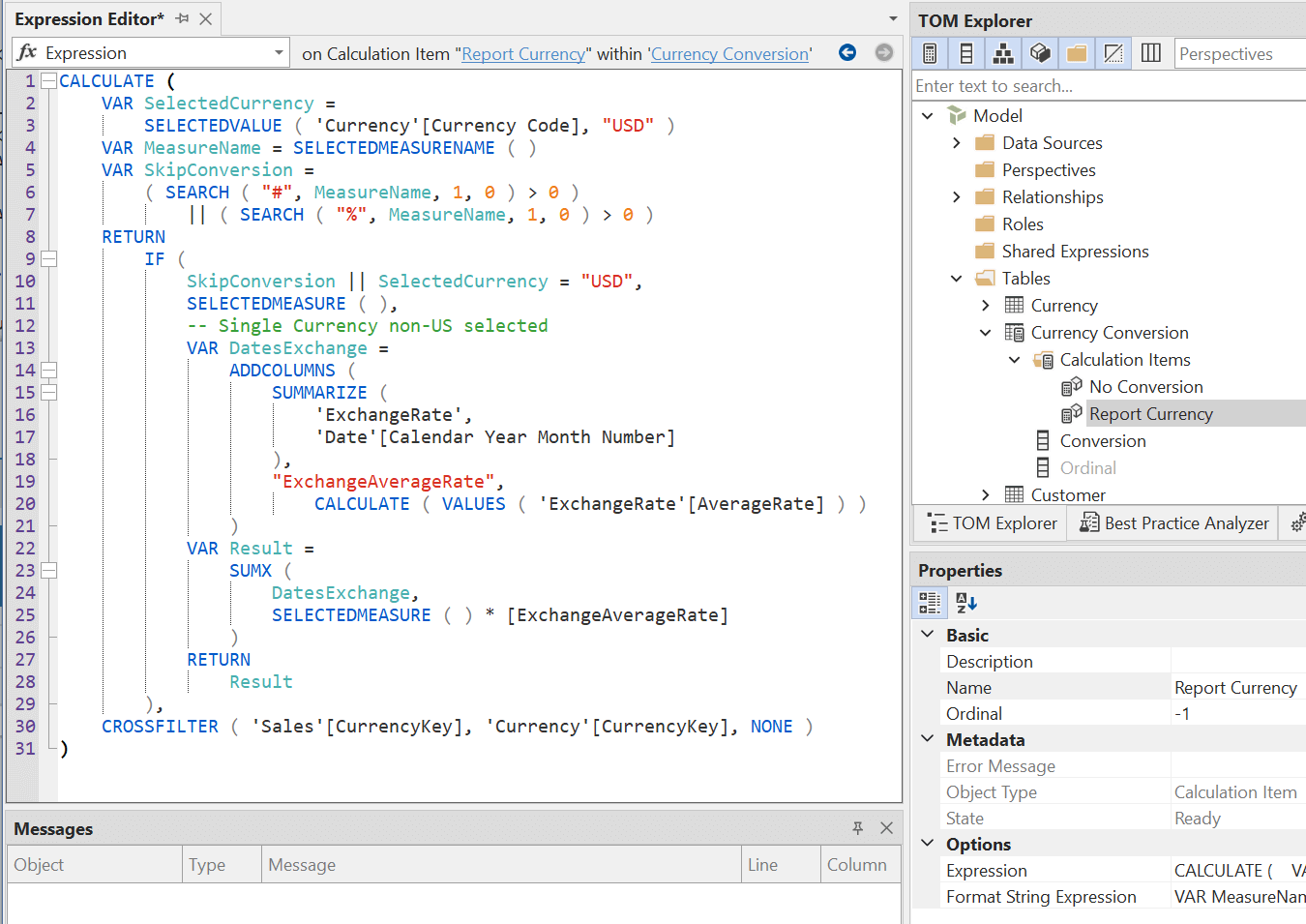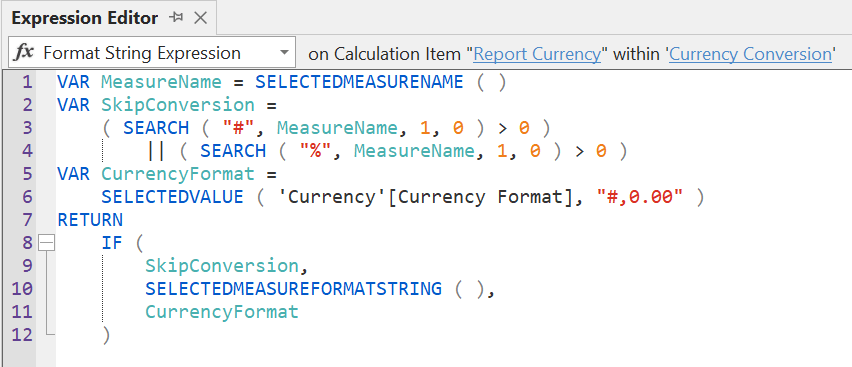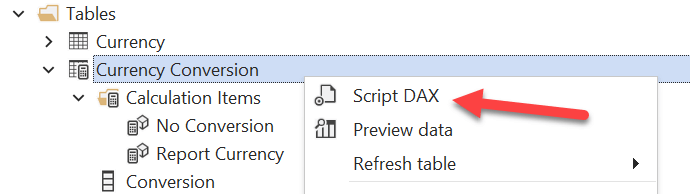When calculation groups were introduced in 2019, we did not have a way to describe them in a textual form. A calculation group was represented as a table with one visible column and one or more rows, one for each calculated item. Each calculation item could have one or two DAX expressions associated with it – one for the calculation item itself and an optional one for the format string. Describing a calculation group in an article often required the writer to include screenshots of the Tabular Editor user interface, plus comments in the sample code to explain where each DAX expression should be placed in the user interface.

From the start we proposed a syntax to describe an entire calculation group in a textual form. However, there was no tool able to convert that syntax into the actual object in the Tabular model. For this reason, in the initial version of the articles about calculation groups we used a “pseudo-syntax” and we included comments that made the code more verbose and not necessarily easier to read. However, Tabular Editor 3 introduced the full DAX script syntax for calculation groups that we had hoped would be available in 2019. We decided to adopt that syntax in our content. We use this article as a guide to introduce and explain the DAX Script syntax for calculation groups.

Before introducing the syntax, let us review the classic representation of a calculation group in Tabular Editor; the user interface is somewhat similar in Visual Studio. In the following screenshot, the Currency Conversion calculation group is represented as a table with two columns: Conversion (visible) and Ordinal (hidden). The content of the Conversion column is defined by the list of Calculation Items. The Report Currency calculation item selected has an Ordinal value of -1 and an expression represented in the Expression Editor.The Format String Expression for the Report Currency calculation item is visible in the next screenshot. The Format String Expression might be empty, in which case the calculation item does not apply any transformation to the format string of the intercepted measure.In order to generate the corresponding script in DAX, we can use the Script DAX context menu item for the Currency Conversion calculation group.The result is the following script. The script includes the definition of both calculation items No Conversion and Report Currency in the Currency Conversion calculation group, which contains a single visible column named Conversion.

Calculation Group
```CALCULATIONGROUP 'Currency Conversion'[Conversion]
Precedence = 30

CALCULATIONITEM "No Conversion" = SELECTEDMEASURE()

CALCULATIONITEM "Report Currency" =
CALCULATE (
VAR SelectedCurrency =
SELECTEDVALUE ( 'Currency'[Currency Code], "USD" )
VAR MeasureName = SELECTEDMEASURENAME ( )
VAR SkipConversion =
( SEARCH ( "#", MeasureName, 1, 0 ) > 0 )
|| ( SEARCH ( "%", MeasureName, 1, 0 ) > 0 )
RETURN
IF (
SkipConversion || SelectedCurrency = "USD",
SELECTEDMEASURE ( ),
-- Single Currency non-US selected
VAR DatesExchange =
SUMMARIZE (
'ExchangeRate',
'Date'[Calendar Year Month Number]
),
"ExchangeAverageRate",
CALCULATE ( VALUES ( 'ExchangeRate'[AverageRate] ) )
)
VAR Result =
SUMX (
DatesExchange,
SELECTEDMEASURE ( ) * [ExchangeAverageRate]
)
RETURN
Result
),
CROSSFILTER ( 'Sales'[CurrencyKey], 'Currency'[CurrencyKey], NONE )
)
FormatString =
VAR MeasureName = SELECTEDMEASURENAME ( )
VAR SkipConversion =
( SEARCH ( "#", MeasureName, 1, 0 ) > 0 )
|| ( SEARCH ( "%", MeasureName, 1, 0 ) > 0 )
VAR CurrencyFormat =
SELECTEDVALUE ( 'Currency'[Currency Format], "#,0.00" )
RETURN
IF (
SkipConversion,
SELECTEDMEASUREFORMATSTRING ( ),
CurrencyFormat
)
```

The first line uses the keyword CALCULATIONGROUP to define the name of the calculation group and the visible column name, followed by optional calculation group properties. The example uses only the Precedence property:

• Description
• Visible
• Precedence

After this, there are one or more CALCULATIONITEM statements. Each statement specifies the calculation item name using a string literal followed by the assignment operator and the DAX expression for the calculation item. This mandatory part can be followed by optional calculation item properties. The example uses FormatString for the Report Currency calculation item:

• Description
• Ordinal
• FormatString

The complete syntax supported for calculation groups is available in the documentation of the DAX Scripting feature.

Using this syntax at SQLBI in our content pertaining to Calculation Groups can simplify the reading. Indeed, the syntax provides all the DAX expressions and properties of a calculation group in a single place that is easier to copy and paste.